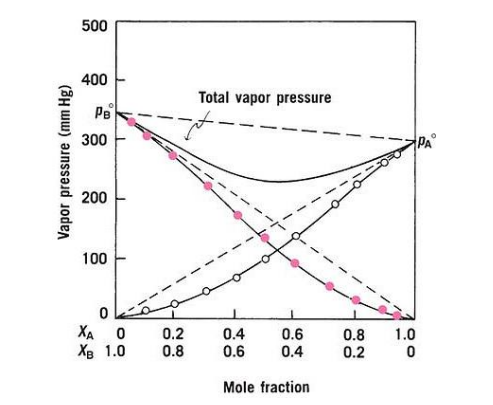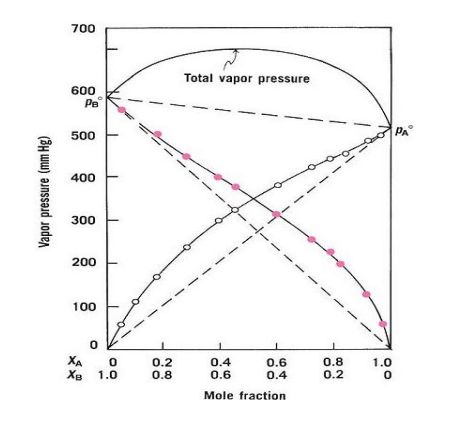Raoult’s Law and Real Solutions : Pharmaguideline -->
 Editable Pharmaceutical Documents in MS-Word Format

# Raoult’s Law and Real Solutions

Chemist François-Marie Raoult discovered, through an experiment conducted in 1877, that when mixed substances were put into a solution.

## Raoult’s law

Chemist François-Marie Raoult discovered, through an experiment conducted in 1877, that when mixed substances were put into a solution, it simultaneously decreased the vapor pressure of the solution. Raoult proposed a law to explain this phenomenon, called Raoult's law, that became part of thermodynamic law.

It can be seen from Raoult's law that the partial vapor pressure in a solution of a pure solvent is equal to the mole fraction of the solvent within the solution multiplied by the vapor pressure. This means that a solution containing mole fractions of a solute will have a lower and higher freezing and boiling point than one containing pure solvents.

Raoult's law equation can be expressed mathematically as follows.

Resolution= Xsolvent.P0Solvent

Where,

Resolution= The solution pressure is equal to the vapor pressure

Xsolvent= A solvent's mole fraction is defined as X solvent

P0Solvent = Pressure at which the pure solvent vaporizes

### Importance

Imagine that a closed container of volatile liquid A is filled. The evaporation of A will result in the formation of vapor particles. In time, there will be a dynamic equilibrium between the vapor particles of A and the liquid particles of the surface. Vapour pressure corresponds to the force exerted by A at a specific temperature by its vapor particles. Solids and liquids exhibit vapor pressure, which is dependent on both the liquid type and its temperature.

Taking this solution as an example, when another liquid B is added to it, the B particles will fill the space between the A particles on the surface of the solution. Molecular energy is sufficient to escape molecules of any given liquid whose surface is in the vapor phase. The number of A vapor particles in the vapor phase should be lower at this point on the surface due to fewer A particles. As a consequence, A's vapor pressure will be lower. In this case, if B is also volatile, there will be fewer particles of B in the vapor phase than there would be in pure liquid B. According to Raoult's law, the new pressure is the partial pressure of A and B in the liquid phase, and it is calculated based on the concentration of each component.

PA ∝ XA , PB ∝ XB = XAP’A= XBP’B

The mole fraction P' is the mole fraction of the constituents

### Limitations

In addition to the limitations of Raoult's Law, there are several others.

As Raoult's law describes ideal solutions, that is, those in which the gas phase exhibits thermodynamic properties comparable to a mixture of ideal gases, it is especially appropriate. The only problem is that they are rare and difficult to locate. There has to be chemical equivalence between different chemical components.

Many liquid mixtures have different attractive forces, so these types of solutions deviate from Raoult's law or do not follow it appropriately or they deviate from Raoult's law.

### Deviations from Raoult’s law

If there is an adhesive force or cohesive force between two liquids, Raoult's Law may have deviated.

#### Negative deviation

Vapour pressures below what is expected are considered negative deviations. A force between particles that is stronger than the force between particles in pure liquids causes this phenomenon. In acetone and chloroform, for example, this behavior is observed. There is a deviation in this mixture because of hydrogen bonds. Hydrochloric acid in solution in water is one example of this.

#### Positive deviation

If cohesion between molecules is greater than the adhesion between unlike molecules, the deviation will be positive. This results in a higher vapor pressure than expected. When the components of the mixture are mixed, they escape the solution more readily than when they are pure. The same behavior is observed in benzene-methanol mixtures as well as in chloroform-ethanol mixtures.

## Real solutions

Raoult's law has deviated in two different ways for the real solution, namely by a positive deviation and a negative deviation. Using Raoult's ideal solution law, vapor pressure ought to be lower than expected when the "adhesive" attraction is greater between molecules than the cohesive attraction between them. When this occurs, there is a negative deviation from the ideal solution. As can be seen in Figure 1, where A is chloroform and B is acetone, the total vapor pressure curve shows a minimum where the deviation is sufficiently large. It is due to the formation of a hydrogen bond between chloroform and acetone that both exhibit this attraction, thus reducing each one's tendency to escape. In the presence of this pair, a weak compound is formed that can be isolated and identified, [Cl3C-H...O=C(CH3)2]. Negative deviations may be caused by reactions between dipolar molecules, or by reactions between dipolar and nonpolar molecules. It is rare, however, to be able to isolate definite compounds in these cases since the interaction is usually so weak.Figure - 1

As A molecules interact less than between pure constituent molecules in the presence of B molecules, the interaction of B molecules between A molecules is reduced, whereas the interaction between A molecules is reduced in the presence of B molecules. Due to their dissimilarities in polarity or pressure, the molecules A and B have a greater tendency to escape from one another. Positive deviations are those that result from partial vapor pressures exceeding those predicted by Raoult's law; thus, partly vaporized constituents experience higher partial vapor pressures than expected. A large deviation in composition can easily lead to the total vapor pressure being at its maximum at one composition. As an example, here is a figure that shows a positive deviation. Benzene and ethyl alcohol, carbon desulfurization and acetone, and chloroform and ethyl alcohol display positive deviations from the norm.Figure - 2

Consequently, Raoult's law does not hold in the entire concentration range of a non-ideal solution. Solvent behavior of actual liquid pairs is only observed if that substance is present in high concentrations, and in such a case, the solvent is the substance that describes the behavior of a real liquid pair. By Raoult's law,

Psolvent = PO solvent Xsolvent

### Henry's law

It is the mole fraction multiplied by the characteristic proportionality constant of the solute that determines the vapor pressure of a volatile solute

Psolute =KsoluteXsolute

Henry's law states that in real liquid pairs diluted solutions, solutes respond to Henry's law, and solvents respond to Raoult's law.
Get subject wise printable pdf documentsAnkur Choudhary is India's first professional pharmaceutical blogger, author and founder of pharmaguideline.com, a widely-read pharmaceutical blog since 2008. Sign-up for the free email updates for your daily dose of pharmaceutical tips.

## Popular Categories

QA SOPs QC SOPs Micro SOPs HVAC Production SOPs Stores SOPs Checklists Maintenance SOPs HPLC Sterile GLP Validation Protocols Water System GDP Regulatory Maintenance Calibration Warning Letters Education B.Pharmacy
Online Courses

## DOCUMENTS

PHARMACEUTICAL DOCUMENTS

Editable Pharmaceutical Documents in MS-Word Format. Ready to use SOPs, Protocols, Master Plans, Manuals and more...

View